Resource Title search

Text Search of NRICH resource titles

Or find resource begining with: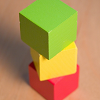3 Block Towers Poster

Age 5 to 7 Challenge Level:

3 Block Towers - July 2010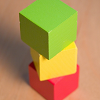3 Blocks Towers

Age 5 to 7 Challenge Level:

Take three differently coloured blocks - maybe red, yellow and blue. Make a tower using one of each colour. How many different towers can you make?3 Rings

Age 7 to 11 Challenge Level:

If you have three circular objects, you could arrange them so that they are separate, touching, overlapping or inside each other. Can you investigate all the different possibilities?3-4-5 Circle

Age 14 to 16 Short Challenge Level:

Can you find the radius of the circle inscribed inside a '3-4-5 triangle'?3-sided

Age 14 to 16 Short Challenge Level:

How many triangles can Jack draw with two sides of lengths $6$cm and $8$cm and an area of $7$cm$^2$?30-60-90 Polypuzzle

Age 16 to 18 Challenge Level:

Re-arrange the pieces of the puzzle to form a rectangle and then to form an equilateral triangle. Calculate the angles and lengths.3388

Age 11 to 14 Challenge Level:

Using some or all of the operations of addition, subtraction, multiplication and division and using the digits 3, 3, 8 and 8 each once and only once make an expression equal to 24.354972

Age 11 to 14 Short Challenge Level:

What is the remainder when 354972 is divided by 7?365 Penguins

Age 5 to 7 Challenge Level: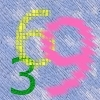396

Age 14 to 16 Challenge Level:

The four digits 5, 6, 7 and 8 are put at random in the spaces of the number : 3 _ 1 _ 4 _ 0 _ 9 2 Calculate the probability that the answer will be a multiple of 396.3d Drawing

Age 11 to 16

How do you represent a three dimensional object on paper?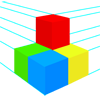3D Drawing

Age 11 to 16

The design technology curriculum requires students to be able to represent 3-dimensional objects on paper. This article introduces some of the mathematical ideas which underlie such methods.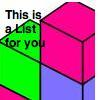3D Investigations

Age 7 to 14 Challenge Level:

A collection of 5 different investigations.3D Shapes3D Shapes

Working on these problems will help you develop a better understanding of three dimensional shapes.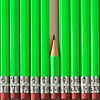3D Shapes - Short Problems

Age 11 to 16

A collection of short problems on 3D shapes.3D Stacks

Age 7 to 14 Challenge Level:

Can you find a way of representing these arrangements of balls?3rd STEM TI Day 2011/12

Age 11 to 18

Resources from the third STEM TI day, June 2012.3rd Stemnrich T I Day 4 July 201

Age 11 to 18 Challenge Level:3x3 Areas

Age 11 to 14 Short Challenge Level:

Weekly Problem 24 - 2007
Which of the following shaded regions has an area different from the other shaded regions?